Creating d3 Components with Angular

@AysegulYonet
AnnieCannons.com

Slides: bit.ly/AngularU-d3

What is a component?

Components = controllers + scopes + directives

What is a d3 Angular component?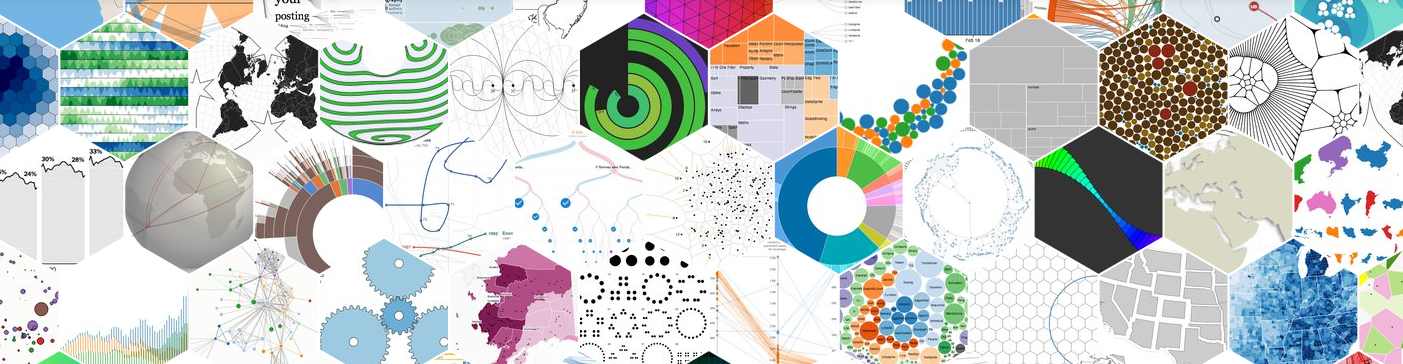“The Angular way” of integrating D3?

• D3 logic in a directive
• Use HTML-declarative syntax to feed of data to your directive instances
• Store the data in your controller
• Create Angular filters using D3 methods.

How we use it in html

``````

``````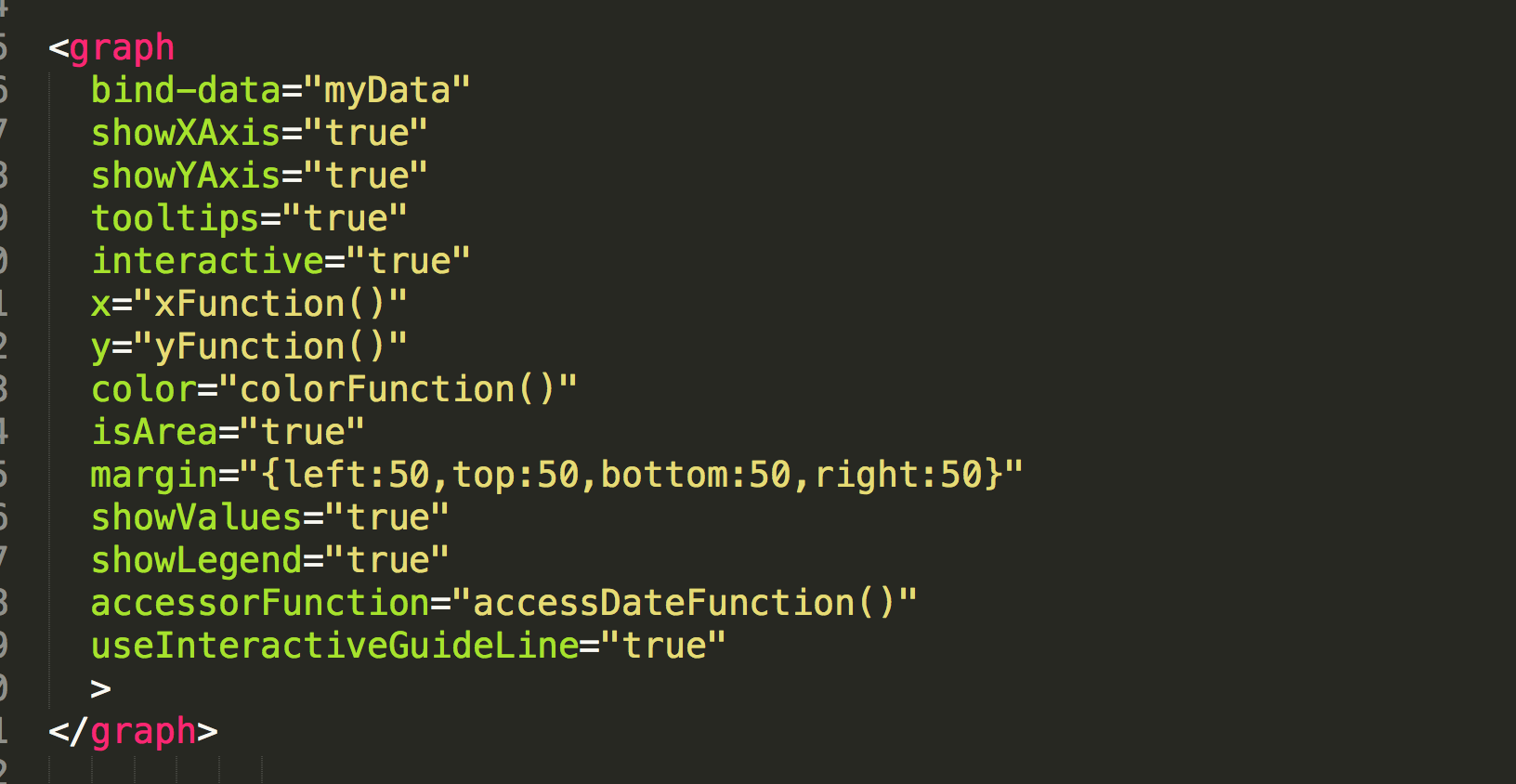``````

``````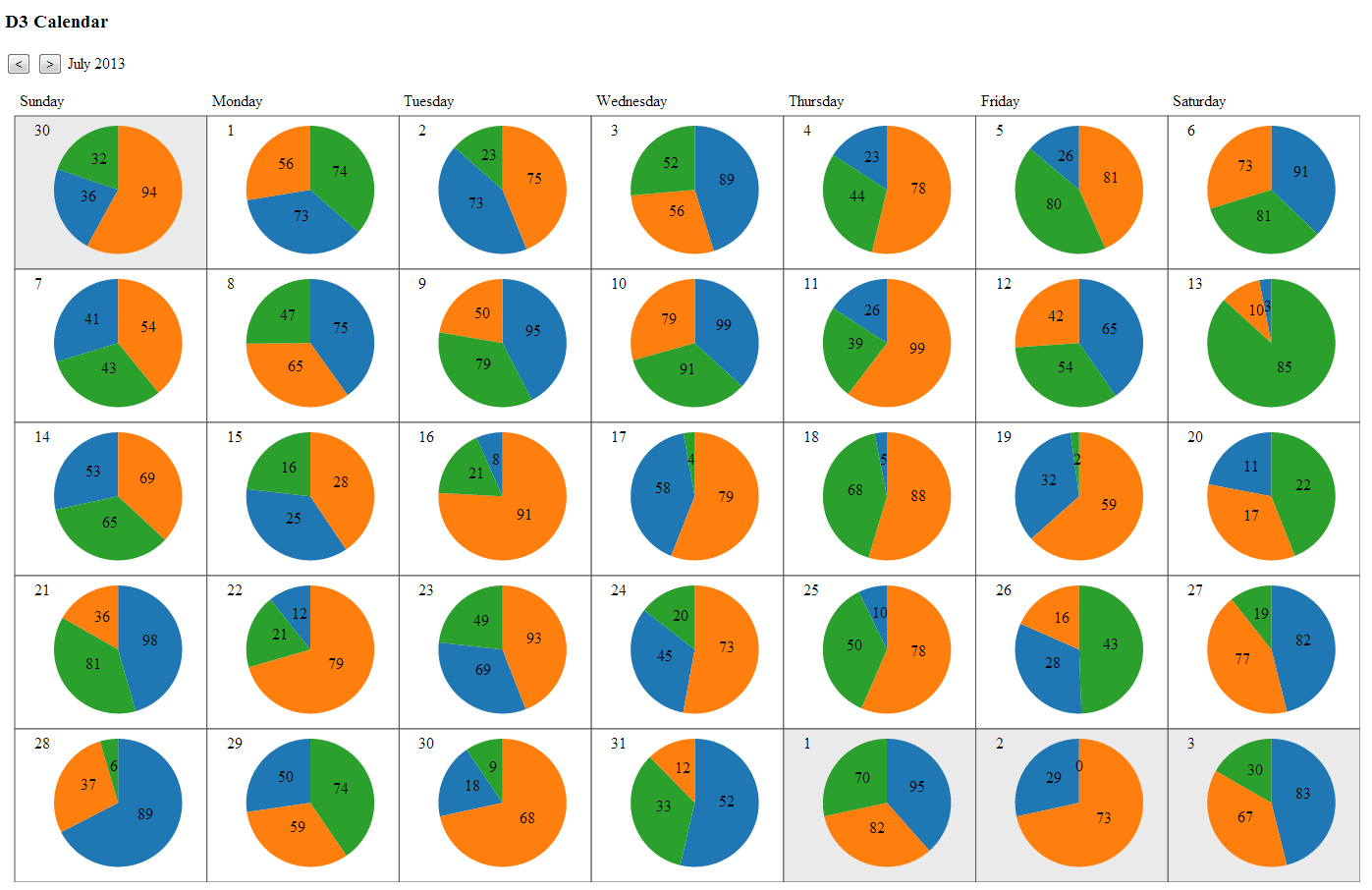how we define Angular 1

``````
angular.module('App', [])
.directive('graph', function() {

return {
restrict: 'E',
scope: {
data:   '=bindData', // bindings
width:  '@width',    // static value
color: '&color'    // expression
},
};

function Graph(scope, element, attrs) { // d3 code
};

});
``````
JsFiddle

Enter and Exit

``````

scope.\$watch('data', function(data){
if (!data) return;
//divs selection with the data
divs = divs.data(data);

//remove the extra elements
divs.exit().remove();
divs.enter().append('div')
.transition().ease("elastic")
.style("width", function (d) {
return d + "%";
})
.text(function (d) {
return d + "%";
});
}, true);

``````

Getting Data

``````
d3.json('data.json', function(err, data){
//render the graph with the data
});
d3.csv('data.csv', function(err, data){...})
``````
``````
\$http.get('/yourApiUrl').
success(function(response) {

// when the response is available
\$scope.data = response.data;
}).
error(function(response) {

});
``````

Example
Array Functions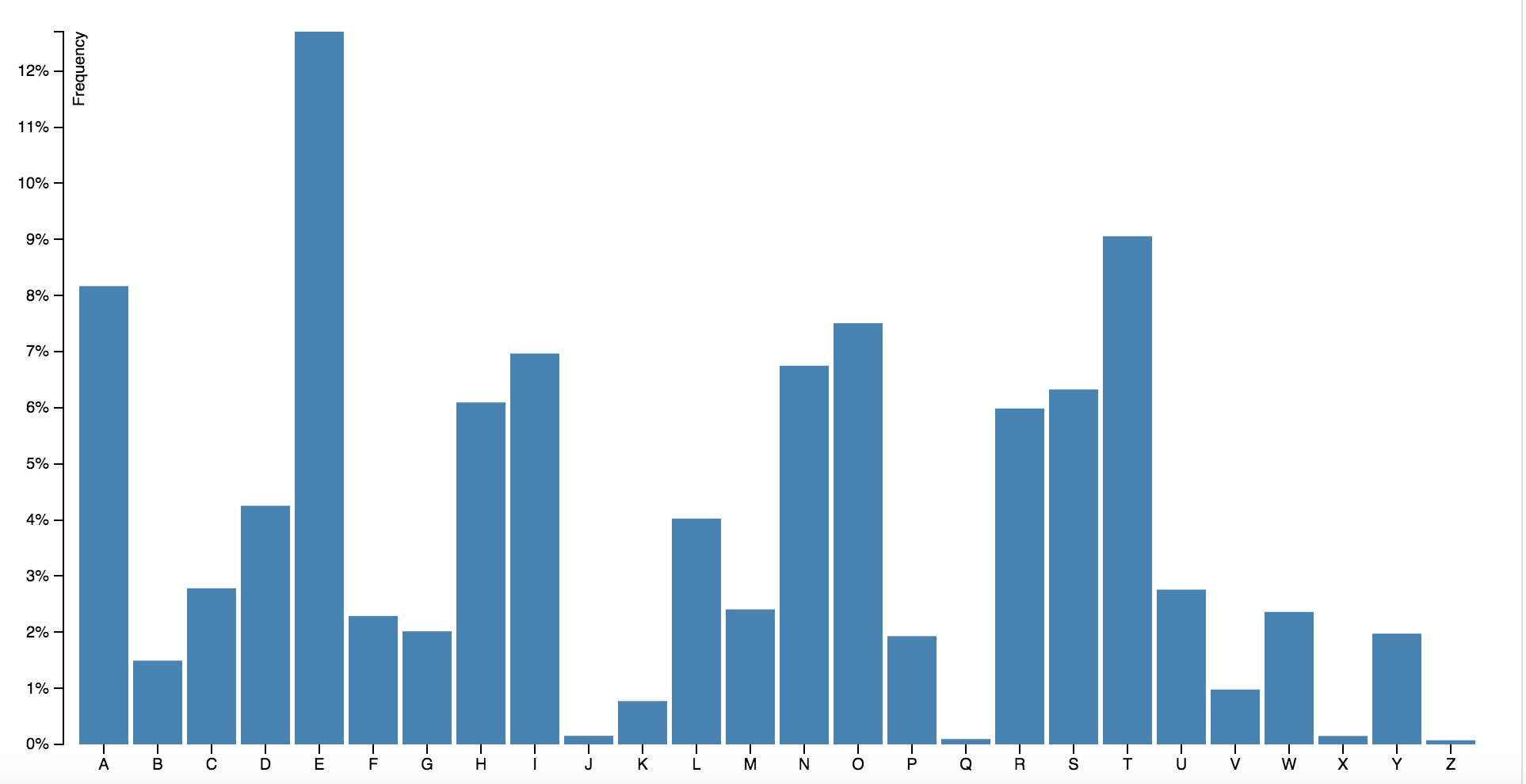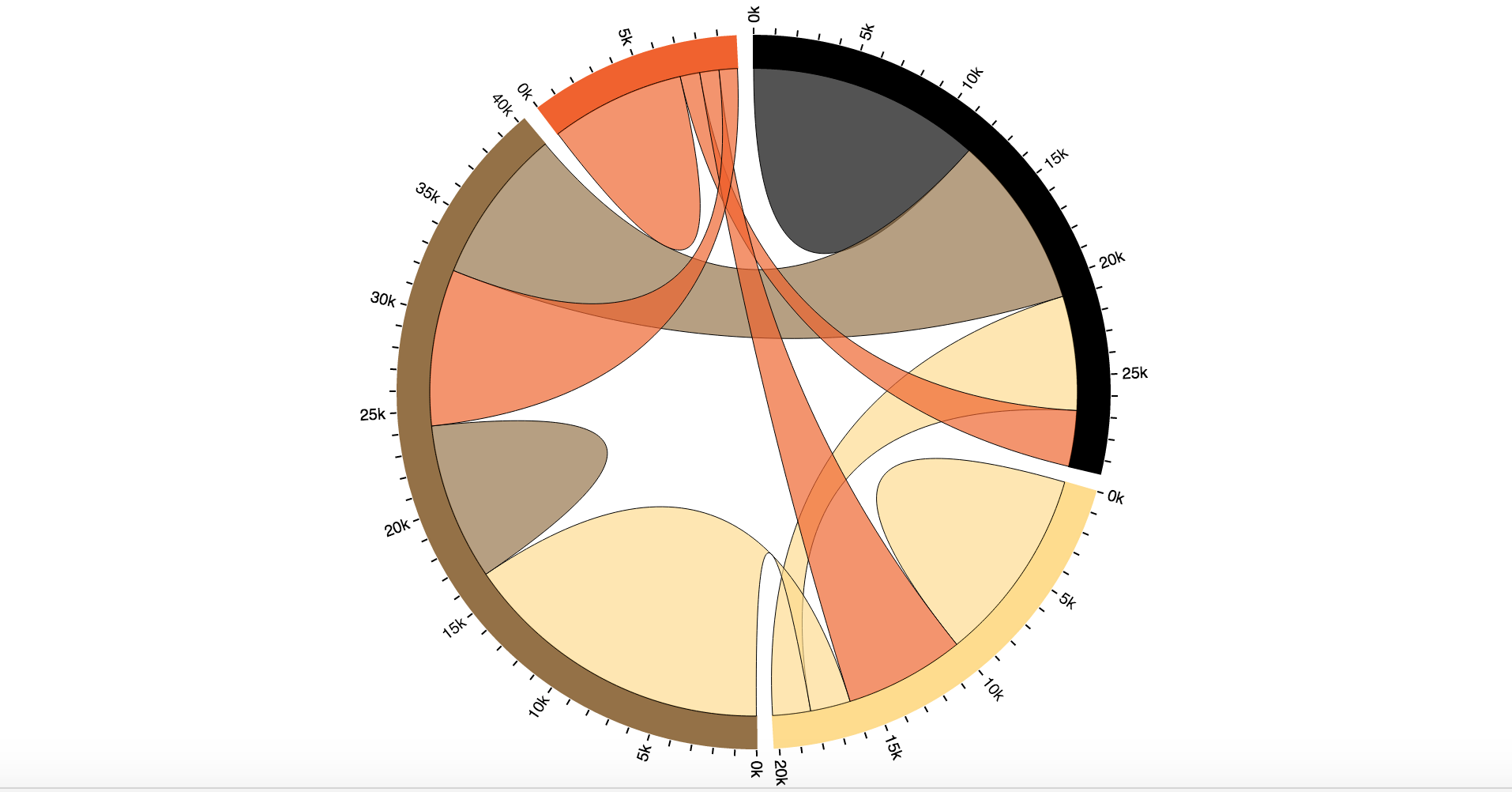D3 array methods

``````

divs.style("width", function (d) {
return scope.accessor({d:d}) + "%";
})
``````
Accessor JSFiddle

TypeScript

``````
npm install -g tsd
tsd query angular2 --action install
``````

``````
npm install -g typescript@^1.5.0-beta
tsc --watch -m commonjs -t es5 --emitDecoratorMetadata app.ts
``````

Using TypeScript

``````
module graphs {

export function graph(): ng.IDirective {
return {
link: (\$scope: ng.IScope, element: JQuery, attributes: any) => {
...construction function goes here.
}
};
}
}
``````

Angular 2

``````
///
import {Component, View, bootstrap} from 'angular2/angular2';
``````

Defining a component

``````
@Component({
selector: 'bar-graph'
})
``````

``````
@Component({
selector: 'bar-graph'
})
@View({
templateUrl: 'path.html',
directives: []
})
class BarGraph {
...
}
``````

``````
bootstrap(BarGraph);
``````

``````

System.import('app');

``````

Service Class

``````
@Component({
...
appInjector: [DataService]
})
class Graph {
data: Array;
constructor(dataService: DataService) {
this.data = dataService.values;
}
}
class DataService {
this.values = [1,2,3];
}
``````

Events

``````

{{newdata.value}}
``````

3D Scatter Plot
More d3 Examples

Thank you!

@AysegulYonet
aysegul@anniecannons.com

Slides: bit.ly/AngularU-d3